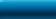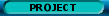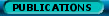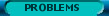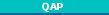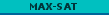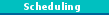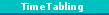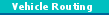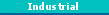MAX-SAT

# MAX-SAT

edited by Thomas Stützle and Luis Paquete, INTELLEKTIK

## Contents

Given n binary variables x(j), j in N, m clauses C_i, i in M, and weights w(i), where N={1,2,... ,n} and M={1,2, ...,m}, the MAX-SAT problem asks to determine a binary tuple (that is, a 0-1 assignment to each of the binary variables) that maximizes the sum of the weights of the satisfied clauses. Each clause is a disjunction of literals l*(j), where a literal is either a variable x(j) (that is, the variable occurs in positive form) or its negation neg x(j) (that is, the variable occurs in negative form). Without loss of generality we assume that at most one of x(j) and neg x(j) is included in each clause. A clause is satisfied if at least one of the positive variables contained in the clause is assigned the value 1 (true) or a negated variable is assigned the value 0 (false).

Given a set of clauses, MAX-SAT is the problem to find a variable assignment that maximizes the weight of satisfied clauses. Note that alternatively, the objective function could have been defined to minimize the weight of the unsatisfied clauses, as it is often done in the literature.

If all the weights are equal to one, we call the resulting problem unweighted MAX-SAT, otherwise we speak of weighted MAX-SAT or simply MAX-SAT. The unweighted MAX-SAT is important, because a special case is the SAT problem. The SAT problem is a decision problem that asks whether a binary tuple can be found that satisfies all clauses (corresponding to a solution of weight n). The SAT problem is a central problem in theoretical computer science, artificial intelligence, mathematical logic, and many applications.

R. Battiti and M. Protasi. Reactive search, a history-based heuristic for MAX-SAT. ACM Journal of Experimental Algorithmics, 2, 1997.

R. Battiti and M. Protasi. Solving MAX-SAT with non-oblivious functions and history-based heuristics. In D. Du, J. Gu, and P.M. Pardalos, editors, Satisfiability problem: Theory and Applications, volume~35 of DIMACS Series on Discrete Mathematics and Theoretical Computer Science, pages 649--667. American Mathematical Society, 1997.

A. Bertoni, P. Campadelli, M. Carpentieri, and G. Grossi. A genetic model: Analysis and application to MAXSAT. Evolutionary Computation, 8(3):291--309, 2000.

B. Borchers and J. Furman. A two-phase exact algorithm for MAX-SAT and weighted MAX-SAT problems. Journal of Combinatorial Optimization, 2(4):299--306, 1999.

P. Hansen and B. Jaumard. Algorithms for the maximum satisfiability problem. Computing, 44:279--303, 1990.

P. Hansen, B. Jaumard, N. Mladenovic, and A.D. Parreira. Variable neighbourhood search for maximum weighted satisfiability problem. Technical Report G-2000-62, Les Cahiers du GERAD, Group for Research in Decision Analysis, 2000.

Y. Jiang, H. Kautz, and B. Selman. Solving problems with hard and soft constraints using a stochastic algorithm for MAX-SAT. In Proceedings of the 1st International Joint Workshop on Artificial Intelligence and Operations Research, 1995.

S. Joy, J. Mitchell, and B. Borchers. A branch and cut algorithm for MAX-SAT and weighted MAX-SAT. In D. Du, J. Gu, and P.M. Pardalos, editors, Satisfiability problem: Theory and Applications}, volume 35 of DIMACS Series on Discrete Mathematics and Theoretical Computer Science, pages 519--536. American Mathematical Society, 1997.

D. McAllester, B. Selman, and H. Kautz. Evidence for invariants in local search. In Proceedings of the 14th National Conference on Artificial Intelligence, pages 321--326. AAAI Press / The MIT Press, Menlo Park, CA, USA, 1997.

P. Mills and E. Tsang. Guided local search for solving SAT and weighted MAX-SAT problems. In I.P. Gent, H. van Maaren, and T. Walsh, editors, SAT2000 --- Highlights of Satisfiability Research in the Year 2000, pages 89--106. IOS Press, Amsterdam, The Netherlands, 2000.

M.G.C. Resende, L.S. Pitsoulis, and P.M. Pardalos. Approximate solution of weighted MAX-SAT problems using GRASP. In D. Du, J. Gu, and P.M. Pardalos, editors, Satisfiability problem: Theory and Applications, volume 35 of DIMACS Series on Discrete Mathematics and Theoretical Computer Science, pages 393--405. American Mathematical Society, 1997.

A. Roli and C. Blum. Critical parallelization of local search for MAX-SAT. In Procedings of AI*IA, 7th Congress of the Italian Association of Artificial Itnelligence, 2001.

B. Selman, H.A. Kautz, and B. Cohen. Noise strategies for improving local search. In Proceedings of the 12th National Conference on Artificial Intelligence, pages 337--343. AAAI Press / The MIT Press, Menlo Park, CA, USA, 1994.

B. Selman, H. Levesque, and D. Mitchell. A new method for solving hard satisfiability problems. In Proceedings of the 10th National Conference on Artificial Intelligence, pages 440--446. AAAI Press / The MIT Press, Menlo Park, CA, USA, 1992.

Y. Shang and B.W. Wah. Discrete lagrangian-based search for solving MAX-SAT problems. In Proceedings of the 15th International Joint Conference on Artificial Intelligence, volume 1, pages 378--383. Morgan Kaufmann Publishers, San Francisco, CA, USA, 1997.

M. Yagiura and T. Ibaraki. Efficient 2 and 3-flip neighborhoods seach algorithms for the MAX SAT. In W.-L. Hsu and M.-Y. Kao, editors, Computing and Combinatorics, volume 1449 of Lecture Notes in Computer Science, pages 105--116. Springer Verlag, Berlin, Germany, 1998.

M. Yagiura and T. Ibaraki. Analyses on the 2 and 3-flip neighborhoods for the MAX SAT. In Journal of Combinatorial Optimization}, 3:95--114, 1999.Network Coordinator: Marco Dorigo    e-mail: mdorigo@ulb.ac.be    Web site responsibles: Christian Blum and Max Manfrin